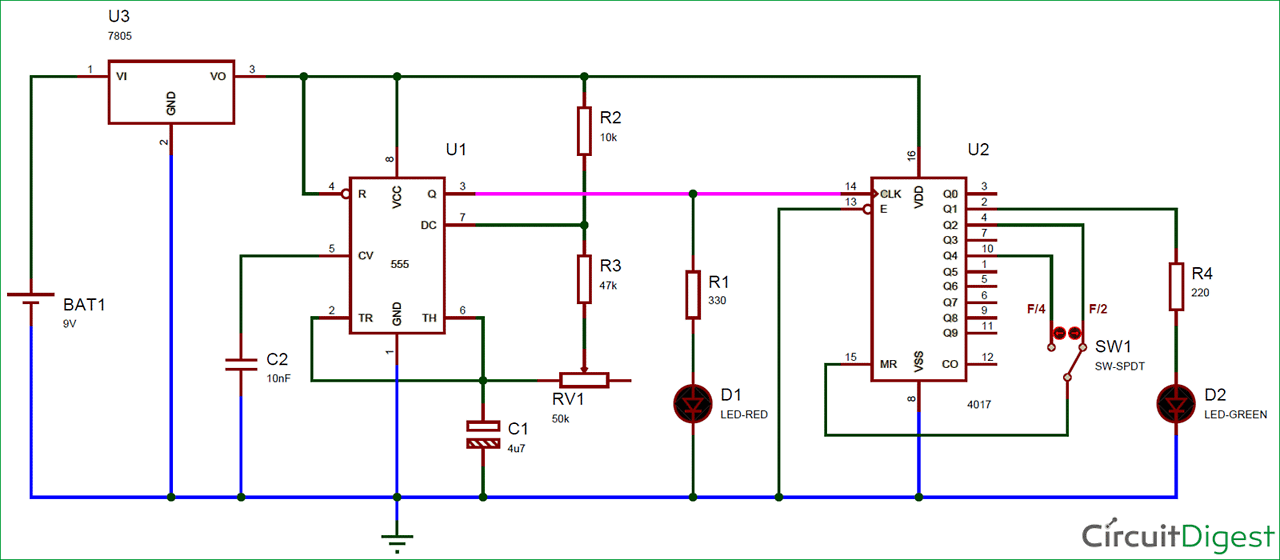# Frequency Divider Circuit## Frequency Divider Circuit

Frequency Dividers are the circuits which divide the input frequency by n (any integer number), means if we provide some signal of frequency ‘f’ then the output will be the divided frequency ‘f/n’. Frequency dividers are very useful in analog as well as digital applications. Here we are building the circuit to divide the frequency by 2 or 4.